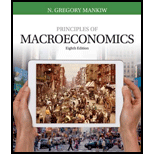# Suppose an economy is in long-run equilibrium. a. Use the model of aggregate demand and aggregate supply to illustrate the initial equilibrium (call it point A). Be sure to include both short-run and long-run aggregate supply. b. The central bank raises the money supply by 5 percent. Use your diagram to show what happens to output and the price level as the economy moves from the initial to the new short-run equilibrium (call it point B). c. Now show the new long-run equilibrium (call it point C). What causes the economy to move from point B to point C? d. According to the sticky-wage theory of aggregate supply, how do nominal wages at point A compare to nominal wages at point B? How do nominal wages at point A compare to nominal wages at point C? e. According to the sticky-wage theory of aggregate supply, how do real wages at point A compare to real wages at point B? How do real wages at point A compare to real wages at point C? f. Judging by the impact of the money supply on nominal and real wages, is this analysis consistent with the proposition that money has real effects in the short run but is neutral in the long run?### Principles of Macroeconomics (Mind...

8th Edition
N. Gregory Mankiw
Publisher: Cengage Learning
ISBN: 9781305971509

#### Solutions

Chapter
Section### Principles of Macroeconomics (Mind...

8th Edition
N. Gregory Mankiw
Publisher: Cengage Learning
ISBN: 9781305971509
Chapter 20, Problem 3PA
Textbook Problem
2767 views

## Suppose an economy is in long-run equilibrium. a. Use the model of aggregate demand and aggregate supply to illustrate the initial equilibrium (call it point A). Be sure to include both short-run and long-run aggregate supply. b. The central bank raises the money supply by 5 percent. Use your diagram to show what happens to output and the price level as the economy moves from the initial to the new short-run equilibrium (call it point B). c. Now show the new long-run equilibrium (call it point C). What causes the economy to move from point B to point C? d. According to the sticky-wage theory of aggregate supply, how do nominal wages at point A compare to nominal wages at point B? How do nominal wages at point A compare to nominal wages at point C? e. According to the sticky-wage theory of aggregate supply, how do real wages at point A compare to real wages at point B? How do real wages at point A compare to real wages at point C? f. Judging by the impact of the money supply on nominal and real wages, is this analysis consistent with the proposition that money has real effects in the short run but is neutral in the long run?

Subpart (a):

To determine
Long run equilibrium in AD-AS model.

### Explanation of Solution

The supply depends upon the price level in the economy. When the price level is higher, the suppliers will be receiving higher income and this would incentivize them to increase the supply in the economy and vice versa. The aggregation of the supply curves of all the firms in the economy is known as the aggregate supply curve. In the short run period, the aggregate supply curve represents the relationship between the price level in the economy and the supply by the firms.

The demand comes from all the economic agents such as the households, firms as well as the government. The demand depends on the price level of the economy. The increase and decrease in the price level determines the level of demand in the economy...

Subpart (b):

To determine
Long run equilibrium in AD-AS model.

Subpart (c):

To determine
Long run equilibrium in AD-AS model.

Subpart (d):

To determine
Long run equilibrium in AD-AS model.

Subpart (e):

To determine
Long run equilibrium in AD-AS model.

Sub part (f):

To determine
Long run equilibrium in AD-AS model.

### Still sussing out bartleby?

Check out a sample textbook solution.

See a sample solution

#### The Solution to Your Study Problems

Bartleby provides explanations to thousands of textbook problems written by our experts, many with advanced degrees!

Get Started